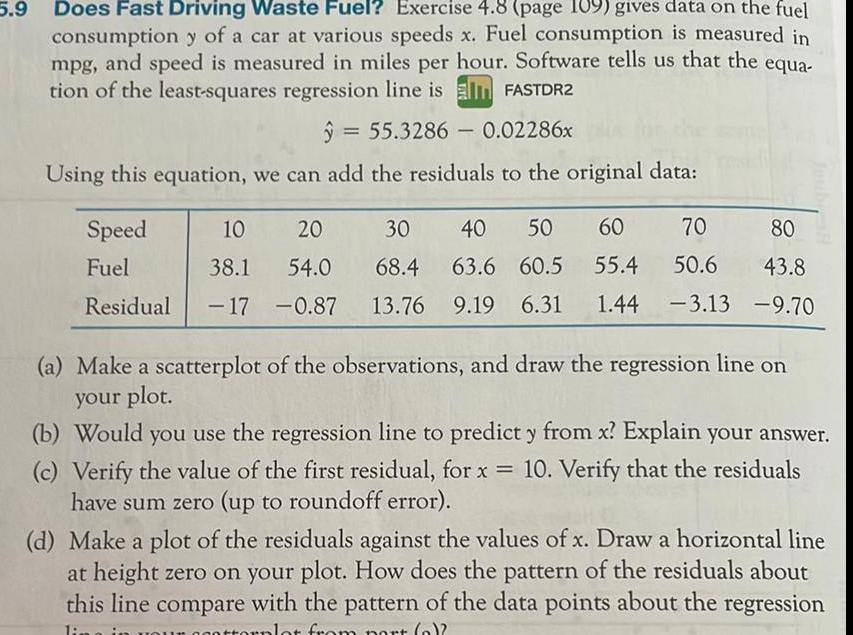Question:

# 5 9 Does Fast Driving Waste Fuel Exercise 4 8 page 109 gives

Last updated: 9/17/20235 9 Does Fast Driving Waste Fuel Exercise 4 8 page 109 gives data on the fuel consumption y of a car at various speeds x Fuel consumption is measured in mpg and speed is measured in miles per hour Software tells us that the equa tion of the least squares regression line is FASTDR2 55 3286 0 02286x Using this equation we can add the residuals to the original data Speed Fuel Residual 10 20 38 1 54 0 17 0 87 80 30 40 50 60 70 68 4 63 6 60 5 55 4 50 6 13 76 9 19 6 31 1 44 3 13 9 70 43 8 a Make a scatterplot of the observations and draw the regression line on your plot b Would you use the regression line to predict y from x Explain your answer c Verify the value of the first residual for x 10 Verify that the residuals have sum zero up to roundoff error d Make a plot of the residuals against the values of x Draw a horizontal line at height zero on your plot How does the pattern of the residuals about this line compare with the pattern of the data points about the regression lina torplot from nort a